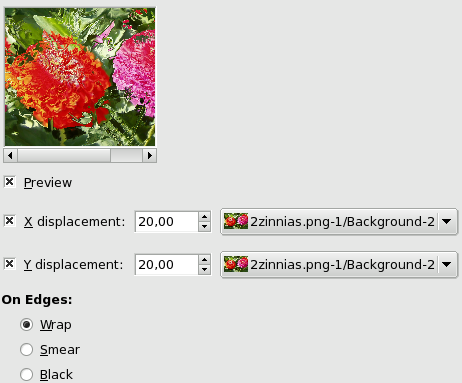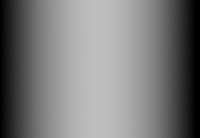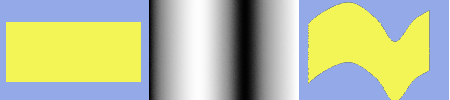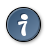### 12.3.  Displace

Revision History
Revision \$Revision: 1985 \$ 2007-07-23 ude

#### 12.3.1.  Overview

Figure 15.199.  Displacement examplesX displacement coefficient is 30 (with a negative coefficient displacement would be inverse). Vacated pixels are black. The displacement map has four grey stripes with values of 210, 160, 110, and 60, respectively.

The image areas corresponding to light gray (≥128) were displaced 19 and 8 pixels to the left. The image areas corresponding to dark gray (≤127) were displaced 4 and 15 pixels to the right.

You can find this filter through FiltersMapDisplace

This filter uses a “displace-map” to displace corresponding pixels of the image. This filter displaces the content of the specified drawable (active layer or selection) by the amounts specified in X and Y Displacement multiplied by the intensity of the corresponding pixel in the 'displace map' drawables. Both X and Y displace maps should be gray-scale images and have the same size as the drawable . This filter allows interesting distortion effects.

#### 12.3.2.  Options

Figure 15.200.  OptionsPreview

Uncheck this option if your processor is slow.

X/Y Displacements

When you select one or both options, active layer pixels corresponding to pixels in the displacement map will be displaced horizontally (X) or/and vertically (Y). Both direction and amount of displacement depend on the intensity of the corresponding pixels in the displacement map.

The map, which must be a grayscale image, has 256 gray levels (0-255). The (theoretical) average value is 127.5. The filter displaces image pixels corresponding to pixels with values less than 127.5 (0 to 127) in the map, to the right for X and downwards for Y. It moves pixels corresponding to pixels with values from 128 to 255 to the left for X and upwards for Y.

Input boxes

X/Y Displacement” should be called X/Y Displacement Coefficient. What you enter in input boxes, directly or by using arrow-head buttons, is not the actual displacement. This coefficient is used in a displacement = (intensity x coefficient) formula which gives the pixel actual displacement according to the scaled intensity of the corresponding pixel in map, modulated by the coefficient you enter. Introducing intensity into formula is important: this allows progressive displacement by using a gradient map.

This value varies in limits equal to the double of image dimensions.

This value may be positive or negative. A negative displacement is reverse of a positive one.

Selecting displacement maps

When you click on the drop-down list button, a list appears where you can select a displacement map. To be present in this list, an image must respect two conditions. First, this image must be present on your screen when you call filter. Then, this image must have the same dimensions as the original image. Most often, it will be a duplicate original image, which is transformed to grey scale and modified appropriately, with a gradient. It may be possible to use RGB images, but color luminosity is used making result prevision difficult. Map may be different in horizontal and vertical directions.

On Edges

These options allows you to set displacement behaviour on active layer or selection edges:

• Wrap: With this option, what disappears on one edge reappears on the opposite edge.

• Smear: With this option, pixels vacated by displacement are replaced with pixels stretched from the adjacent part of the image.

• Black: With this option, pixels vacated by deplacement are replaced with black.

#### 12.3.3.  Using gradient to bend a text

2. Duplicate this image. Activate this duplicate and make it gray-scaled (<IMAGE>/Image/Mode/GrayScale). Fill it with the wanted gradient. This image will be your Displacement map, with the dimensions of original image.3. Activate original image. Create a Text Layer with your text. Set layer to image size: right-click on the layer in layer dialog and, in the pop-menu, click on “Layer to image size”. Note that letters in text layer lie on a transparent background; now this filter doesn't displace transparent pixels. Only letters will be displaced.4. Activate text layer. Open Displace filter window. Set parameters particularly displacement coefficient according to the result in Preview. OK.This method also applies to standard layers:TipTo get the wanted gradient, first draw a black to white gradient. Then use the Curves tool to modify the gradient curve.

#### 12.3.4.  Displacement Calculation

The following section will show you how to calculate the amount of displacement, if you are interested in these details. If you don't want to know it, you can safely omit this section.

The overview example showed the X displacement using a coefficient of 30.0: 19, 8, 4, or 15 pixels, depending on the grey level of the displacement map's color.

Why just these amounts? That's easy:If you check these equations, you will notice that the values they give are not exactly the results we retained in the example (using non-integers, that's not surprising). So, were the results rounded to the nearest integer and then the pixels were displaced by a whole-numbered amount? No. Every pixel is displaced exactly by the calculated amount; a “displacement by a fractional amount” is realized by interpolation. A closer look at the example image will show it:

Figure 15.201.  A closer look at the displacement exampleA small area zoomed in by 800 percent.

The displacement causes small (one pixel wide) areas of intermediate colors at the edges of plain color areas. E.g., the black area (zoomed in image) is caused by a displacement of -4.12, so the intermediate color is 12% black and 88% gold.

So if you select a displacement coefficient of 30.01 instead of 30.00, you will indeed get a different image, although you won't see the difference, of course.

 Scaled intensity = (intensity - 127.5) / 127.5; see section “displacement calculation”.